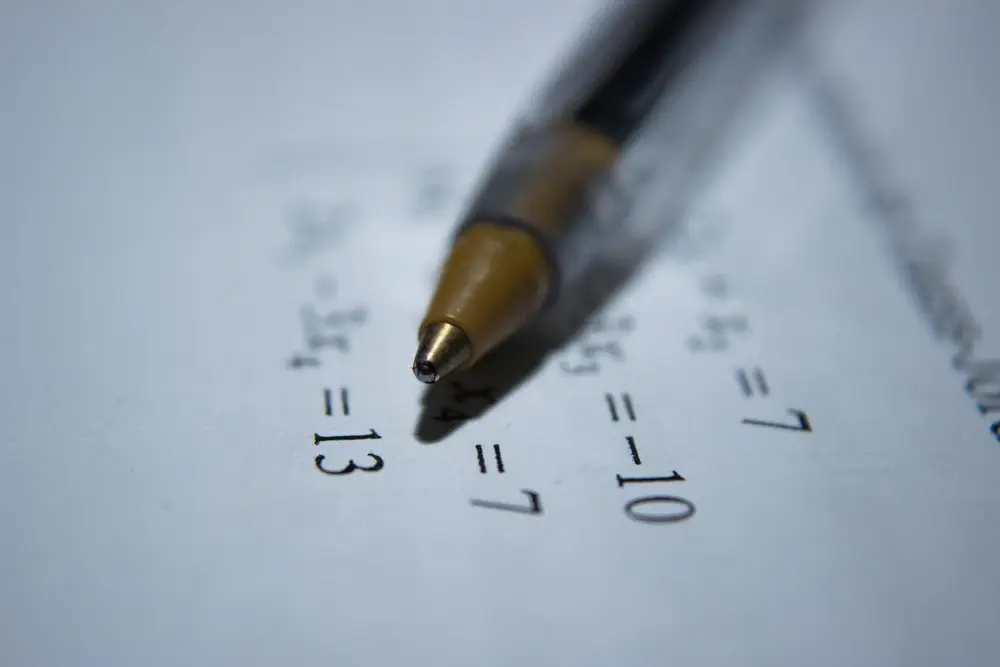# Simultaneous Equations

Example:
A man buys 3 fish and 2 chips for £2.80
A woman buys 1 fish and 4 chips for £2.60
How much are the fish and how much are the chips?

There are two methods of solving simultaneous equations. Use the method which you prefer.

Method 1: elimination
First form 2 equations. Let fish be f and chips be c.
We know that:
3f + 2c = 280 (1)
f + 4c = 260 (2)
Doubling (1) gives:
6f + 4c = 560 (3)
(3)-(2) is 5f = 300
so f = 60
Therefore the price of fish is 60p

Substitute this value into (1):
3(60) + 2c = 280
so 2c = 100
c = 50
Therefore the price of chips is 50p

Method 2: Substitution
Rearrange one of the original equations to isolate a variable.
Rearranging (2): f = 260 - 4c
Substitute this into the other equation:
3(260 - 4c) + 2c = 280
so 780 - 12c + 2c = 280
so 10c = 500
so c = 50
Substitute this into one of the original equations to get f = 60 .

Harder simultaneous equations:
To solve a pair of equations, one of which contains x², y² or xy, we need to use the method of substitution.
Example:
2xy + y = 10 (1)
x + y = 4 (2)
Take the simpler equation and get y = .... or x = ....
from (2), y = 4 - x (3)
this can be substituted in the first equation. Since y = 4 - x, where there is a y in the first equation, it can be replaced by 4 - x .
sub (3) in (1), 2x(4 - x) + (4 - x) = 10
so 8x - 2x² + 4 - x - 10 = 0
so 2x² - 7x + 6 = 0
so (2x - 3)(x - 2) = 0
so either 2x - 3 = 0 or x - 2 = 0
therefore x = 1.5 or 2 .

Substitute these x values into one of the original equations.
When x = 1.5, y = 2.5
when x = 2, y = 2

Simultaneous equations can also be solved by graphical methods.

Algebra

Algebra

Algebra

Algebra

Algebra

Algebra